Vibrations Index Page

Forced Vibrations

 Steady State Forced Vibrations On the page   Ref..Forced Vibrations    covering vibration of a mass (m) with a k spring stiffness and c viscous damping subject to a sinusoidal force of P cosω t. The steady state solution for the system was shown to be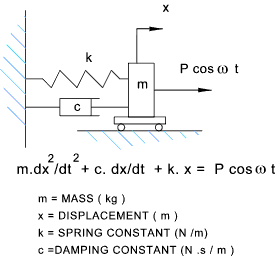x = A cos ( ω t - φ ) The modulus A was shown to be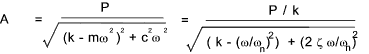The phase angle φ was shown to be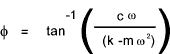using the following derived relationships.. .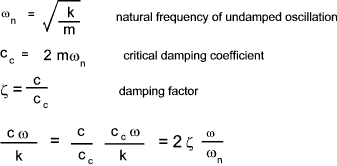The dimensionless relationship between the Static displacement P/k (= Xo )and the Peak amplitude X is provided by the following expression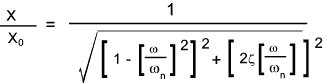The phase angle can be related to the driving frequency and the natural frequency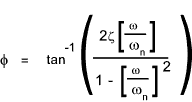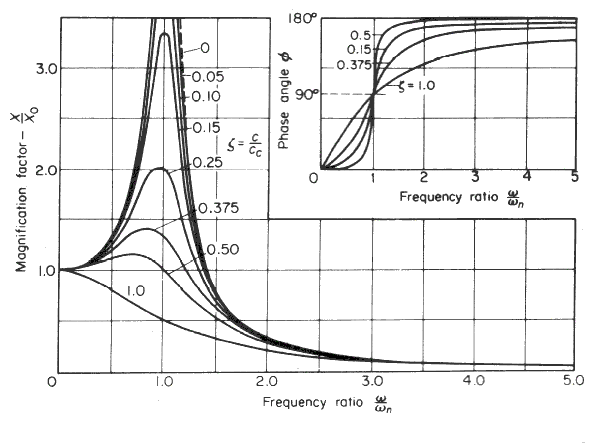Rotating Unbalanced Mass For the case of a mass M supported on springs k with viscous damping c which is supporting a rotating unbalanced load of mass m rotating with and angular frequency ω at a radius r .    The peak amplitude and phase are provided by the following dimensionless expressions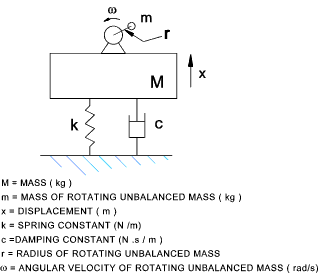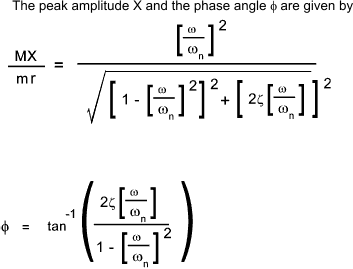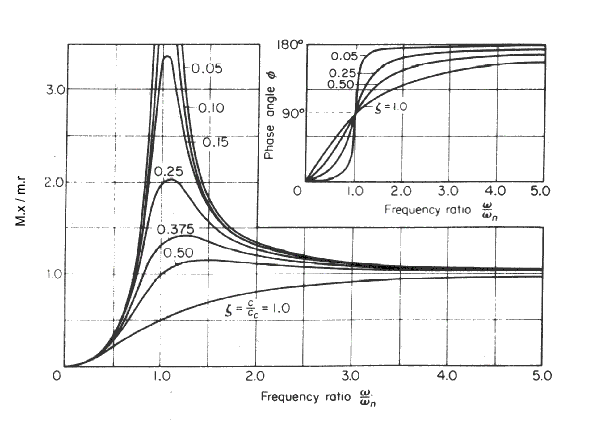Excitation of Base For the case of a mass m supported on springs k with viscous damping c on a base which is moving with an excitation of form y = Y sin ω t . Note: proof may be most easily be obtained from link 3 below;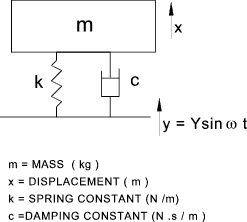The ratio of peak ampltude of the mass and the base and the phase are provided by the following dimensionless expressions Note: The amplitude ratio is also the ratio of the transmitted force to the exciting force it is a measure of the transmissibility of the system � the ratio of the energy going into the system to the energy coming from the system.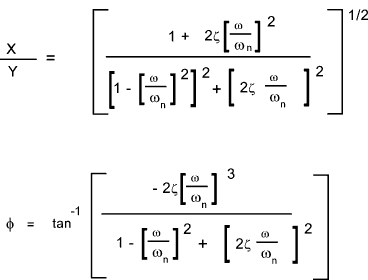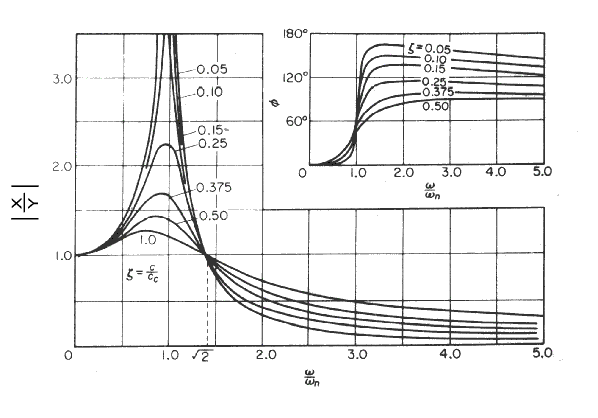Links on Free Vibrations Damped Forced Vibrations Tool...Java applet providing visual display or forced vibrations Theory Of Vibrations...Excellent easy to follow notes EN4 Dynamics and Vibrations...Brown University Lecture Notes -
Vibrations Index Page